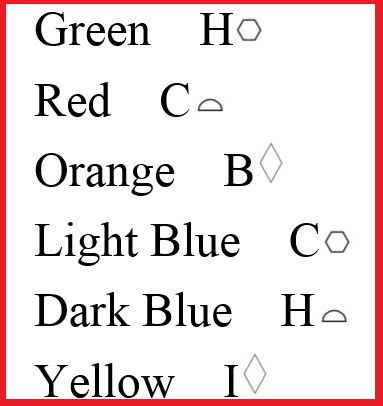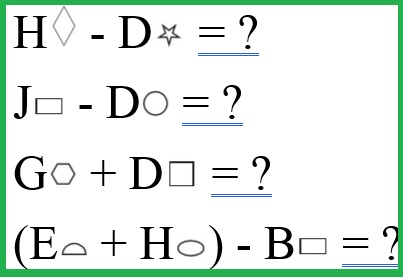# Activity Mats 52 & 53: My Six Bricks Number Grid 2

€0.95
In stock
SKU
SB094

This is a double-sided A3 Gloss Laminated Mat for mathematics. Each individual rectangle is designed to take a ‘Six Brick’ standing vertically.

Side A: My Six Bricks Hundred Grid Blank

Side B: Six Bricks Number Rectangles

The mats target a wealth of number concepts 1 to 100, which follows directly on from ‘My Six Bricks Number Line’ to 10.

Each of the 4 grids has a ‘grid referencing’ system on the periphery allowing every number to be designated a coordinate, which not only adds a layer of complexity but facilitates whole-class correction of tasks. A very clever system, the first of its kind!

We are practising identifying numbers on the blank 100 grid using our Six Bricks.

• Place Green on 1
• Place Red on 10
• Place Orange on 11
• Place Light Blue on 20
• Dark Blue on 80
• Yellow on 99

Children discuss and check their answers (positions of bricks) relative to those of their neighbour.

Note 1: The teacher can also have the children confirm that they have the colours in the correct position by calling out the grid references e.g. Yellow is 99 which is (I, Octagon), Red is 10 (J,Triangle)

Note 2: This task can be repeated over and over for other numbers. Children become very skilled at predicting and working out where numbers are based on their relativity to known numbers and place value understanding.

We are learning to use a ‘Grid Referencing’ system.

Ask the students to place the following bricks upright on the board in the following positions:Next, ask them to write down the six numbers that are covered by each brick, if the grid starts at 1 and finishes at 100.

Green = ?, Red = ?, Orange = ?, Light Blue = ?, Dark Blue = ? and Yellow = ?

Children confer, discuss and check their answers with those of their neighbour.

Note: This task can be repeated over and over for other grid references and numbers.

Finding the following totals: if the value of the first number rectangle is 1:

1. What is the total value of the group of 6 Green Bricks?
2. What is the value of the group of 6 Red Bricks?
3. What is the value of the group of 6 Orange Bricks?
4. What is the value of the group of 6 Light Blue Bricks?
5. What is the value of the group of 6 Dark Blue Bricks?
6. What is the value of the 6 Yellow Bricks?

Solve the following equations:Write the numbers in order as follows

1. Clockwise from bottom right, write the 6 red numbers in order?
2. Clockwise from top left, write the 6 light blue numbers in order?
3. Clockwise from bottom left, write the 8 red numbers in order?
4. Anti-clockwise from top right, what are the 6 green numbers?
5. Clockwise top left, what are the 4 numbers at the centre of green/blue
6. Anti-clockwise bottom right, what are the 4 numbers at the centre of red/blue
7. What is double the numbers at the centre of orange / yellow?